Exponents And Powers - MCQ

# Exponents And Powers - MCQ

Test Description

## 10 Questions MCQ Test Class 8 Mathematics by VP Classes | Exponents And Powers - MCQ

Exponents And Powers - MCQ for Class 8 2022 is part of Class 8 Mathematics by VP Classes preparation. The Exponents And Powers - MCQ questions and answers have been prepared according to the Class 8 exam syllabus.The Exponents And Powers - MCQ MCQs are made for Class 8 2022 Exam. Find important definitions, questions, notes, meanings, examples, exercises, MCQs and online tests for Exponents And Powers - MCQ below.
Solutions of Exponents And Powers - MCQ questions in English are available as part of our Class 8 Mathematics by VP Classes for Class 8 & Exponents And Powers - MCQ solutions in Hindi for Class 8 Mathematics by VP Classes course. Download more important topics, notes, lectures and mock test series for Class 8 Exam by signing up for free. Attempt Exponents And Powers - MCQ | 10 questions in 10 minutes | Mock test for Class 8 preparation | Free important questions MCQ to study Class 8 Mathematics by VP Classes for Class 8 Exam | Download free PDF with solutions
 1 Crore+ students have signed up on EduRev. Have you?
Exponents And Powers - MCQ - Question 1

### Which of the following is the value of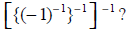Exponents And Powers - MCQ - Question 2

### Which of the following is the value of ‘m’ is 6m ÷ 6– 3 = 65

Exponents And Powers - MCQ - Question 3

### Which of the following is the standard form of 0.00001275?

Exponents And Powers - MCQ - Question 4

Which of the following is the used form of 5 . 05 × 106 ?

Exponents And Powers - MCQ - Question 5

For which of the following m = 8?

Detailed Solution for Exponents And Powers - MCQ - Question 5

Explanation : [(5m)/5-3]/52 = 53

(5m * ⅕-3)/52 = 53

[5m *1/(1/S)3]/52 = 53

[5m *(1/(1/S)2)/(5)^3]/52 = 53

(5m/53)/(52) = 53

(5m/53)* 1/(52) = 53

5m = (53)(53)(52)

5m = 58

m = 8

Exponents And Powers - MCQ - Question 6

1 micron is equal to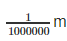. Which of the following is its standard form?

Exponents And Powers - MCQ - Question 7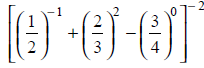is equal to:

Exponents And Powers - MCQ - Question 8

Which of the following is equal to [100 – 990] × 100?

Exponents And Powers - MCQ - Question 9

Which of the following is the reciprocal of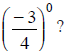Exponents And Powers - MCQ - Question 10

Which of the following is the value of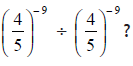## Class 8 Mathematics by VP Classes

89 docs|16 tests
 Use Code STAYHOME200 and get INR 200 additional OFF Use Coupon Code
Information about Exponents And Powers - MCQ Page
In this test you can find the Exam questions for Exponents And Powers - MCQ solved & explained in the simplest way possible. Besides giving Questions and answers for Exponents And Powers - MCQ, EduRev gives you an ample number of Online tests for practice

89 docs|16 tests